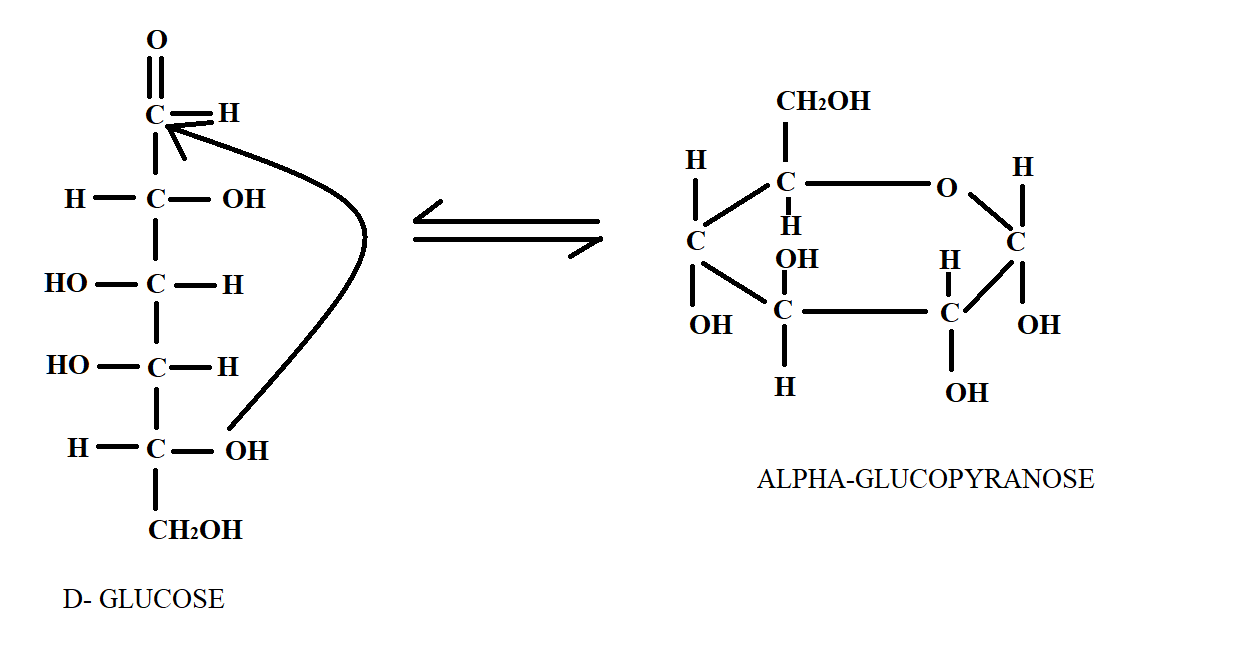Courses
Courses for Kids
Free study material
Free LIVE classes
MoreLIVE
Join Vedantu’s FREE Mastercalss

# Pyranose ring structure of glucose is due to hemiacetal formation between :a.) ${C_1}$ and ${C_5}$b.) ${C_1}$ and ${C_4}$c.) ${C_1}$ and ${C_3}$d.) ${C_2}$ and ${C_4}$Verified
334.5k+ views
Hint : Pyranose is the name of ring structure which has five carbon atoms. The hemiacetal linkage will be between those carbon atoms which will form a five membered ring structure.

We have studied that Glucose is a six carbon long carbohydrate. It is a monosaccharide sugar that can be shown by linear structure or cyclic structure. Pyranose is a general term for any cyclic isomer that has five carbon atoms and one oxygen atom thereby forming a six-membered ring structure. The reversible closure of an open chain monosaccharide to form two anomeric cyclic forms is called mutarotation. It is this phenomenon by which the linear chain of glucose is converted to pyranose form of glucose. This can be seen in the following diagram below.In the above diagram, we see the structure of Glucose in linear chain and its conversion to glucopyranose and now by counting the carbon number; we can find out that hemiacetal linkage occurs at ${C_1}$ and ${C_5}$ positions.

So, option a.) is the correct answer.

Note :
The hemiacetal linkage is the combination of two functional groups. It is an alcohol part and ether part attached with the same carbon atom. It is derived from acetal in which two ether groups are attached with the same carbon atom. Hemi means half. Presence of one group of ether makes it hemi acetal.
Last updated date: 26th Sep 2023
Total views: 334.5k
Views today: 9.34k